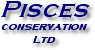Planning: The first step in any study

The Planning function helps you to decide how many samples are required to prove that a difference is significant; 4 methods are available:

• Fisher's Exact - calculates the power of a 2 x 2 contingency table analysed using Fisher's exact test.
• t-Test Unequal Variances - calculates the power for detecting the differences between two means taken from distributions with different variances.
• t-Test Equal Variances - calculates the power for detecting the differences between two means taken from distributions with similar variances.
• Power of Correlation - calculates the power to detect a correlation between two variables.Understanding the sampling requirements is the key to research success -
target your analyses to prove significance